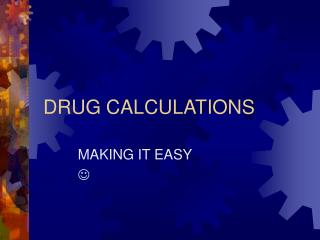DownloadDownload PresentationDRUG CALCULATIONS

# DRUG CALCULATIONS

Télécharger la présentation## DRUG CALCULATIONS

- - - - - - - - - - - - - - - - - - - - - - - - - - - E N D - - - - - - - - - - - - - - - - - - - - - - - - - - -
##### Presentation Transcript

1. DRUG CALCULATIONS MAKING IT EASY 

2. CLASS OUTLINE • 1) review roman numerals • 2) review apothecary measurements • 3) review conversions • 4) review 3 steps to dosage calculations • 5) practice, practice, practice • 

3. Plugging in to Memory • Roman Numerals

4. APOTHECARY MEASURES • What are we talking about?

5. CONVERSIONS • - WITHIN SYSTEMS • - BETWEEN DIFFERENT SYSTEMS

6. WITHIN SYSTEMS • MEMORIZE: • 1 gram (g or G) = 1000 milligrams (mg) • 1 liter (l or L) = 1000 milliliters (ml or mL) • 3 teaspoons (t) = 1 tablespoon (T) • 1 cubic centimeter (cc) = 1 milliliter (ml)

7. BETWEEN SYSTEMS • MEMORIZE: • 1 gram (G, GM, gm) = 15 grains (gr) • 60 milligrams (mg) = 1 grain (gr) • 1 tablespoon (T) = 15 ml • 1 kilogram (kg) = 2.2 pounds (lb) • 1 ounce (oz) = 30 ml or 30 cc • 1 cc = 15 drops (gtt) or 15 minims (mx) • 1 inch (in) = 2.5 centimeters (cm)

8. STEPS TO CONVERSION

9. WITHIN SYSTEMS • 1) Write down what you know in a ratio or fraction format: • Example: 1 Gram • 1000 milligrams

10. 2) Write down what you desire in a ratio or fraction format to complete the proportion: • Example: 3 Grams • x milligrams

11. Remember: • The numerator and denominator must be the same unit of measurement • The unknown value is referred to as “x”

12. The completed proportion: • 1 Gram3 Grams • 1000 mg : : x milligrams • Cross multiply

13. 1 GM x X mg(s) = 3 GM x 1000 mg • Drop the terms used for units of measurement: 1 X = 3(1000) • Solve for X: divide both sides of the equation by the number preceding X: • X = 3000 • 1 1 X = 3000

14. BETWEEN SYSTEMS • 1) Write down what you know in a fraction format: • 15 grains • 1 gram

15. 2) Complete the proportion by writing down what you desire: • 15 grains30 grains • 1 gram : : X grams • HINT: Keep the units of measurements in the correct spaces!

16. Drop the units of measurement • Cross multiply • 15 x X = 30 x 1 • 15X = 30 • Solve for X: Divide each side by the number in front of X : X = 2 ANS: 2 Grams

17. VOILA!!

18. 3 STEPS TO DOSAGE CALCULATION: • 1) Convert to units of same system and similar unit • 2) STOP AND THINK • 3) Desired over amount on hand times quantity • EX: desired • amount on hand x quantity

19. SAMPLE PROBLEMS • 1) Convert: 300 mg to grams • 2) Convert: 120 mg to grains • 3) Convert: 30 gr to grams • 4) A child who weighs 55 pounds weighs ____ • kilograms?

20. 5) A patient is restricted to four 8-ounce glasses of water per day. The nurse knows that the patient’s fluid intake is restricted to ____ mls per day. • 6) The nurse administered aspirin gr v. She knew this was equivalent to _____milligrams(s).

21. 7) Potassium chloride tablets are available in 300 mg doses. The physician prescribed gr v. Give ____ tablet(s). • 8) The physician prescribed Capoten, 1.5 grams daily in three equal doses, for hypertension. The nurse would give _____ 1000 mg tablet(s) three times a day.

22. 9) Lasix 30 mg, IM, is prescribed as a diuretic. Lasix was available as 40 mg/mL. The nurse would give ______mL. • 10) Scopolamine 0.3 mg was ordered subcutaneously as a preanesthetic medication. The medication was available in ampules containing grains 1/200 per mL. The nurse would give _____mL.

23. 11) The physician prescribed 25 mg of Librium, IM. Add 2 mL of diluent to yield 100 mg/2ML. The nurse should give _____ mL. • 12) The physician prescribed 5 mg of Garamycin for a child. The medication is available as 20 mgs/mL. To give 5 mg, you would give ____ mL.

24. PRACTICE, PRACTICE, PRACTICE • And HOLLER if you need help!